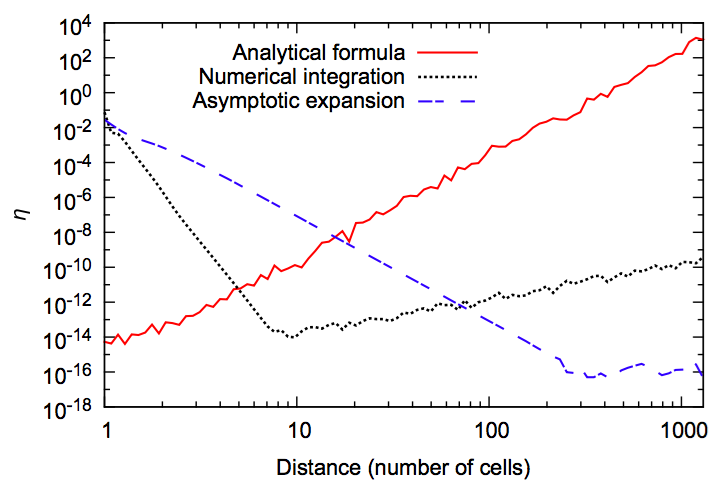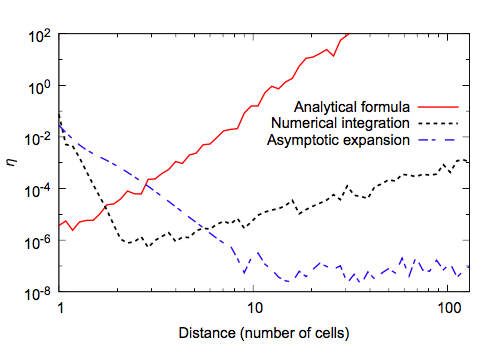# Computing the demagnetizing tensor for finite difference micromagnetic simulations via numerical integration

Summary of Dmitri Chernyshenko's recent publication of more accurate ways of computing the demagnetisation tensor in finite difference micromagnetics.

Dmitri Chernyshenko, and Hans Fangohr
Computing the demagnetizing tensor for finite difference micromagnetic simulations via numerical integration
Journal of Magnetism and Magnetic Materials, 381 440-445 (2015)
(Online: journal, arXiv.org)

## Context: Micromagnetic simulations

Micromagnetic simulations underpin a wide range of research. The list of papers citing the popular OOMMF micromagnetic simulation package approaches 2000 publications.

A wide spread approach to solve the time-dependent non-linear partial differential equations found in micromagntecis is the finite difference method.

The micromagnetic interaction that is computationally hardest to compute is the demagnetisation; this is comparable to bar magnetics interacting with each other: it is a long range interaction.

The demagnetising field (short 'demag' field) computation has to be carried out in every time step (or every energy minimisation iteration). The most common approach in finite difference is to compute a demagnetising tensor, which needs to be convoluted with the (zero padded) magnetisation. This can be done effectively in Fourier space, although the efficient calculation is not relevant for the work discussed here: here, we focus on the accuracy of this demagnetisation tensor.

## Accuracy of the demagnetisating tensor

The demagnetisation tensor can be computed based on the analytical expression for interacting uniformly magnetised cuboids (see Refs [7, 12, 13, 14] in paper). Early work that Michael Donahue presented at a conference (Ref  in paper) shows that this analytical expression becomes increasingly in-accurate the further away the two interacting cuboids are. This is in agreement with our results:Figure 1 [in paper] shows the growing error in the analytical equation (red solid line) as the distance between the cells grows. The x-axis is expressed in multiples of simulation cells, and we can read from the chart that for a spacing of 400 cells, the relative error of the demag tensor approaches 1. In other words, for spacings larger than this, it is more accurate to ignore the demag term than to include it.

Mike Donahue suggested that for large cell distances, one could use the low-order expansion of the full multipole interaction between the two cuboids. This approximation is the more accurate the further away the interacting cells are as higher order multipole moments decay more quickly with distance; for the largest distances the dipole moment will dominate. This can be seen by following the blue dashed line labelled 'asymptotic method' in Figure 1 above.

We can see that there is a cross-over point at around 11 cell spacings where the analytical method (red) and the asymptotic method (blue) have the same errors.

## Main result: new method to compute demag tensor entries more accurate for intermediate distances

The novelty in this work is to provide another recipe to compute the demag tensor entries in a different way. This is based on numerical integration of the equations that lead to the analytical result, and delivers better accuracy for intermediate ranges. This data is shows as the dotted black line labelled 'numerical integration' in figure 1, and is better than the analytical method for cell spacings greater than 5. It is better than the asymptotic method up to about 70 cell spacings.

At first sight, this result may sound surprising: numerical integration appears to be (for intermediate cell distances) more accurate than evaluating the exact solution of the integral.

A separate blog bost on Numerical integration more accurate than the exact result? explains in detail how this can happen in principle for a 1d integral. In short, there is loss of significant digits in evaluation the exact result and this doesn't happen in the numerical integration which is based on additive expressions. While the computation of the demagnetisation tensor involves 6-dimensional integrals, and thus the numerical integration is computationally more challenging than in the 1d toy example, the basic problem and solution idea are identical.

## Other findings

The paper explores a number of different integration schemes: as the integral to solve is 6-dimensional, there is a wide variety of schemes possible and optimising execution speed and accuracy is not trivial.

We further provide data points for the computational effort of computing the demag tensor entries (Table 2) .

We also compute the accuracy characteristics as shown in figure 1 using single floating point numbers (i.e. 4 byte rather than 8 byte per floating point number):Figure 4 [in paper] shows the same data is Figure 1 (see above), but using only single precision floating point numbers.

Using single precision floating point numbers, the analytical expression has an error exceeding 100% for more than 10 cell spacings. Most micromagnetic simulations will have more than 10 cells in some direction, and thus the error of the demag tensor entries exceeds 100% very quickly. It would thus be more accurate not to include the demag term at all, then to rely on an inaccurate tensor. The main conclusion here is that the analytic equation for the demag tensor should be computing using double precision floating point numbers, in particular because it needs to be computed only once at the beginning of the simulation, even if the main computation of the magnetisation field is done in single precision (for example for performance reasons on GPU hardware).

## Summary

• Study accuracy of different methods to compute demag tensor entries for finite difference micromagnetic simulations.
• Analytical expression is most accurate for short distances between cells, and least accurate for large distances.
• Asymptotic expansion of the interaction is most accurate for large distances, and least for smallest.
• New method based on numerical integration gives better accuracy for intermediate distances.

## Full text access and paper details

Dmitri Chernyshenko, and Hans Fangohr
Computing the demagnetizing tensor for finite difference micromagnetic simulations via numerical integration
Journal of Magnetism and Magnetic Materials, 381 440-445 (2015) (Online: <a href="http://www.sciencedirect.com/science/article/pii/S0304885315000153"journal arXiv.org)

PDFs are available from:

## Bibtex entry

``````@article{Chernyshenko2015,
title = "Computing the demagnetizing tensor for finite difference micromagnetic simulations via numerical integration ",
journal = "Journal of Magnetism and Magnetic Materials ",
volume = "381",
number = "0",
pages = "440 - 445",
year = "2015",
note = "",
issn = "0304-8853",
doi = "http://dx.doi.org/10.1016/j.jmmm.2015.01.013",
url = "http://www.sciencedirect.com/science/article/pii/S0304885315000153",
author = "Dmitri Chernyshenko and Hans Fangohr",
arxiv = "http://arxiv.org/abs/1403.1978"
}
``````